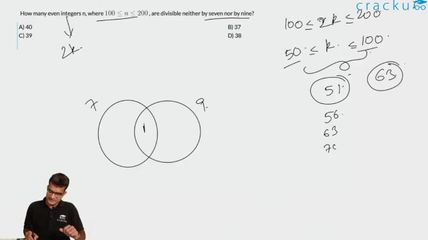Question 65

# How many even integers n, where $$100 \leq n \leq 200$$ , are divisible neither by seven nor by nine?

Solution

Between 100 and 200 both included there are 51 even nos. There are 7 even nos which are divisible by 7 and 6 nos which are divisible by 9 and 1 no divisible by both. hence in total 51 - (7+6-1) = 39

There is one more method through which we can find the answer. Since we have to find even numbers, consider the numbers which are divisible by 14, 18 and 126 between 100 and 200. These are 7, 6 and 1 respectively.

### View Video Solution